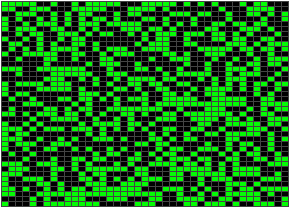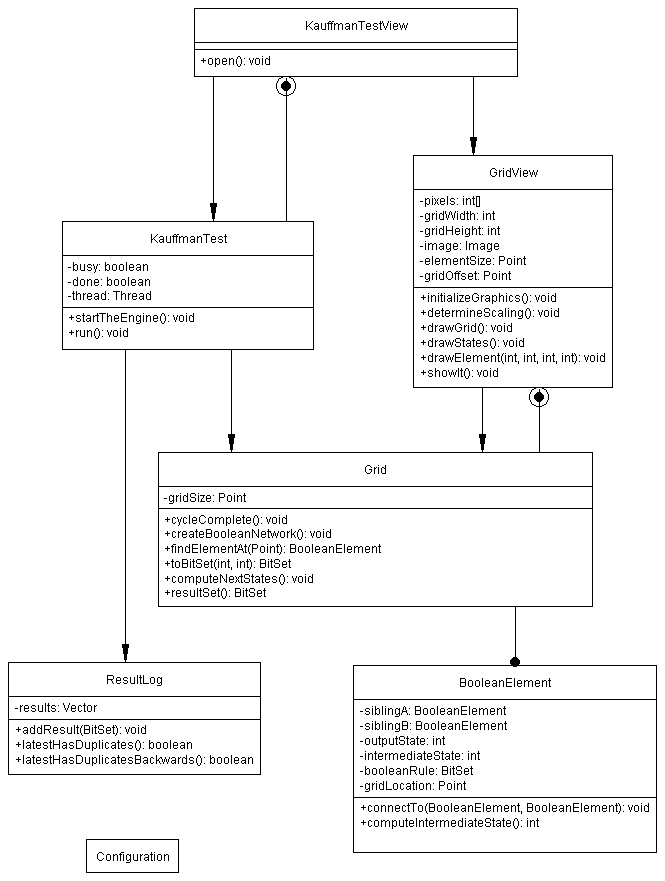OO Programming in Java

Class 7 Material

Kauffman Boolean NetworkProlog

Your boss has asked you to help discover how life got started on earth.

One theory is that molecules in the primordial ooze of primative earth began to form autocatalytic systems, that is systems which locked themselves into stable chemical systems. Chemical A produces Chemical B which produces Chemical C which then produces more of Chemical A.

How easy is it to form these autocatalytic systems? That's what we are trying to find out.

To test this theory your boss has asked you do write a program to implement an artificial chemistry using Kauffman Boolean Networks and see how easily they fall into stable systems. In our artificial chemistry an autocatalytic system would be one where the outputs of all the nodes are identical to a previous time step. Thus we have state A, state B, state C, state ..., state Q, state A, state B ...... There is a 'cycle'.

If there are 1600 interconnected BooleanElements there are 2^1600 possible states (about 10 with 500 zeros after it). The probability of getting a repeat seams quite slim.

Kauffman Boolean Network Basics

A Kauffman Boolean Network is a network of BooleanElement node objects.

A BooleanElement's state is determined by two inputs and by it's boolean rule (AND, OR, etc). Each BooleanElement's rule is randomly determined at initialization. If a particular BooleanElement has inputs 0 and 1, and it's rule is OR, then it's output is a 1.

BooleanElements are connected together to form a network such that each BooleanElement is connected to exactly two others. The state of a BooleanElement's two neighbors provide its inputs and its rule determine its outputs. These networks are allowed to step through states. A network advances to the next state when each BooleanElement reads it neighbors and computes a new output based on its boolean rule.

Quiz

Design an object model and scenario diagram to implement Kauffman Boolean Networks described above.

Object Model